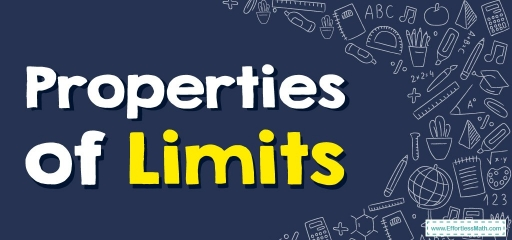# Properties of Limits

Knowing the properties of limits allows us to calculate the limit directly. We can add, subtract, multiply and divide the limits of the functions as if we were performing operations on the functions themselves to find the result.It’s almost time for us to calculate some limits. However, before doing so, we need some properties of limits that make our life somewhat easier. So, let’s look at them first.

## A step by step guide to properties of limits

The following is the list of properties of limits:

We assume that $$lim_{x\to a}f(x)$$ and $$lim_{x\to a}g(x)$$ exist and $$c$$ is a constant. Then,

• $$\color{blue}{lim_{x\to a}[c . f(x)]=c\ lim_{x\to a} f(x)}$$

You can factor a constant that is multiplicative out of a limit.

• $$\color{blue}{lim_{x\to a}[f(x) ± g(x)]=lim_{x\to a}f(x) ± lim_{x\to a}g(x)}$$

To consider the limit of a sum of difference, select the limits individually and put them back with the corresponding sign. This fact works regardless of the number of functions we separated by $$+$$ or $$-$$.

• $$\color{blue}{lim_{x\to a}[f(x) . g(x)]=lim_{x\to a} f(x) . lim_{x\to a} g(x)}$$

Consider product limits similar to sums or differences. just select the limit of the pieces and put them back, and this is not limited to just two functions.

• $$\color{blue}{lim_{x\to a}[\frac{f(x)}{g(x)}]=\frac{lim_{x\to a} f(x)}{lim_{x\to a} g(x)}}$$, $$\color{blue}{lim_{x\to a} g(x) ≠0}$$

we need to bother only if the limit of the denominator is zero when operating the quotient limit. If it were zero, it ends up with a division by zero error.

• $$\color{blue}{lim_{x\to a}[f(x)]^n]=[lim_{x\to a}f(x)]^n}$$, where $$n$$ is any real number

In this property $$n$$ can be any real number (positive, negative, integer, fraction, irrational, zero).

• $$\color {blue}{lim_{x\to a}[\sqrt[n]{f\left(x\right)}]=\sqrt[n]{lim_{x\to a}f(x)}}$$
• $$\color{blue}{lim_{x\to a} c= c}$$

The limit of a constant is only a constant.

• $$\color{blue}{lim_{x\to a}x=a}$$
• $$\color{blue}{lim_{x\to a}x^n=a^n}$$

Note: all these properties also hold for the two one-sided limits as well we just didn’t write them down with one-sided limits to save on space.

### Properties of Limits – Example 1:

Calculate the value of the following limit. $$lim_{x\to -2}(4x^2+3x-2)$$

First, we use this property to break up the limit into three separate limits: $$\color{blue}{lim_{x\to a}[f(x) ± g(x)]=lim_{x\to a}f(x) ± lim_{x\to a}g(x)}$$

$$lim_{x\to -2}(4x^2+3x-2)$$ $$=lim_{x\to -2}4x^2+ lim_{x\to -2}3x- lim_{x\to -2}2$$

Then, use this property to bring the constants out of the first two limits: $$\color{blue}{lim_{x\to a}[c . f(x)]=c\ lim_{x\to a} f(x)}$$

$$=lim_{x\to -2}4x^2+ lim_{x\to -2}3x- lim_{x\to -2}2$$ $$=4lim_{x\to -2}x^2+ 3lim_{x\to -2}x- lim_{x\to -2}-2$$

Now, use these property to solve limit:

$$\color{blue}{lim_{x\to a} c= c}$$ and $$\color{blue}{lim_{x\to a}x^n=a^n}$$

$$=4lim_{x\to -2}x^2+ 3lim_{x\to -2}x- lim_{x\to -2}-2$$

$$=4(-2)^2+3(-2)-2$$

$$=16-6-2=8$$

### Properties of Limits – Example 2:

Calculate the value of the following limit. $$lim_{x\to 3}(4x^2)$$

To find the limit, use this formula: $$\color{blue}{lim_{x\to a}[c . f(x)]=c\ lim_{x\to a} f(x)}$$

$$lim_{x\to 3}(4x^2)$$ $$=4 lim_{x\to 3} x^2$$

$$= 4(3)^2=4(9)$$

$$=36$$

## Exercises for the Properties of Limits

### Calculate the value of the following limit.

1. $$\color {blue}{lim_{x\to 2}(2x+3)^4}$$
2. $$\color{blue}{lim_{x\to 3}\frac{x^2-x-6}{x-3}}$$
3. $$\color{blue}{lim _{x\to 4}\left(\frac{\frac{1}{x}-\frac{1}{4}}{x-4}\right)}$$
4. $$\color{blue}{lim _{x\to 7}\left(\frac{x^2+7x+12}{x+7}\right)}$$
5. $$\color{blue}{lim _{x\to 0}\left(\frac{\sqrt{36-x}-6}{x}\right)}$$
1. $$\color{blue}{2401}$$
2. $$\color{blue}{5}$$
3. $$\color{blue}{-\frac{1}{16}}$$
4. $$\color{blue}{\frac{55}{7}}$$
5. $$\color{blue}{-\frac{1}{12}}$$

### What people say about "Properties of Limits - Effortless Math: We Help Students Learn to LOVE Mathematics"?

No one replied yet.

X
30% OFF

Limited time only!

Save Over 30%

SAVE $5 It was$16.99 now it is \$11.99